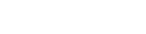# Javascript Array includes()

In JavaScript, we use an array include() function to see if an array contains an element. If an array contains an element, the JavaScript includes() function returns true. Else, false is returned.

## Syntax

``````
array.includes(element, start)

``````
ParameterDescription
elementRequired - The value to look for.
startOptional - Its a start position, default value is 0.

Lets take an example of include function which returns true.

``````
let alphabets = ['A', 'B', 'C', 'D']
console.log(alphabets.includes('B'))

``````
##### Output
``````
true

``````

Lets take an example of include function which returns false.

``````
let alphabets = ['A', 'B', 'C', 'D']
console.log(alphabets.includes('E'))

``````
##### Output
``````
false

``````